# SSD performance - issues again

#### Rand__

##### Well-Known Member
So my next attempt to find a suitable storage for my ESX boxes, this time a large single SSD filer (with a secondary hdd/optane box + smaller slower vsan robo cluster for mixed mode high resilience).

So I restarted my old napp-it installation (Feb '18), ran update (none avail) and the started blasting away at my pool.

1. CPU is a 2667 ES2 (2.6Ghz), 4 cores allocated to the VM on a X10SRA (x16/x8/x8/x8)
2. 16 GB Ram allocated
3. Drives are S3700 400Gb - i have a large number in this system but it didn't look good so I went back to a small pool to check.
4. Disks are in a Supermicro 846 with -A Backplane and attached to a 9305-16i (x8) and a 9305-8i (in x8)
5. All tests local , tried e1000 and vmxnet3 network card even if it should not matter, still old hardware version from ova, ESX is some 6.5 variant

I ran 4,6,8 drives in mirrors - most values are kind of identical and I have no clue why that would be?
Will recheck Bios settings in the morning just to be sure.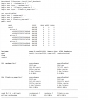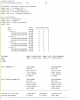#### Evan

##### Well-Known Member
Oh, maybe 20% of performanceSorry can’t really add anything constructive since all looks ok to me setup wise, using controllers in the correct slots, setup seems ok.

Last edited:

#### Rand__

##### Well-Known Member
At least the dd tests are better than what I see, but haven't run those explicitly yet.

#### gea

##### Well-Known Member
Something is limiting the performance as there is nearly no increase over number of mirrors. I would rule out now possible reasons ex

- compare a barebone setup (rule out ESXi)
- compare Sata (AHCI) results of a Raid-10 (rule out 9305 HBA)

Then motherboard related things like
- reset bios to defaults
- remove half of RAM, then check with with other half, optionally different RAM types

- try a different mainboard

update:
I have just seen that you have set readcache=none. Such a setting will extremely reduce performance as you operate like a system with 1-2 GB RAM and this is only usefull if you want to compare 2 settings and want to rule out RAM effects (RAM is what makes ZFS fast)

Compare a readcache=all (default setting for regular operation)

Last edited:

#### gea

##### Well-Known Member
Depends on what you want to know.
If you want to know the real difference between a disk, a SSD and an Optane NVMe you want to rule out RAM effects as a workload that can be processed in RAM is nearly as fast on any of them.

If you want to know how fast is a given system, you want to include RAM in the benchmark.

#### Rand__

##### Well-Known Member
So, tried installing OmniOS baremetal but that didnt want to work via my KVM switch. Couldnt create a bootable USB stick from it iso eitherWent with a linux install instead and ran fio (1 and 16 iodepth):

Code:
``````Fio X10SRA Native, R1, SATA, E52667v4qs

root@ubuntusra:/root#  fio --randrepeat=1 --ioengine=libaio --direct=1 --gtod_reduce=1 --name=test --

test: (g=0): rw=randwrite, bs=4K-4K/4K-4K/4K-4K, ioengine=libaio, iodepth=1
fio-2.2.10
Starting 1 process
Jobs: 1 (f=1): [w(1)] [100.0% done] [0KB/94212KB/0KB /s] [0/23.6K/0 iops] [eta 00m:00s]
test: (groupid=0, jobs=1): err= 0: pid=1734: Wed May  2 15:22:43 2018
write: io=8192.0MB, bw=93215KB/s, iops=23303, runt= 89992msec
cpu          : usr=5.03%, sys=22.28%, ctx=2097286, majf=0, minf=9
IO depths    : 1=100.0%, 2=0.0%, 4=0.0%, 8=0.0%, 16=0.0%, 32=0.0%, >=64=0.0%
submit    : 0=0.0%, 4=100.0%, 8=0.0%, 16=0.0%, 32=0.0%, 64=0.0%, >=64=0.0%
complete  : 0=0.0%, 4=100.0%, 8=0.0%, 16=0.0%, 32=0.0%, 64=0.0%, >=64=0.0%
issued    : total=r=0/w=2097152/d=0, short=r=0/w=0/d=0, drop=r=0/w=0/d=0
latency   : target=0, window=0, percentile=100.00%, depth=1

Run status group 0 (all jobs):
WRITE: io=8192.0MB, aggrb=93215KB/s, minb=93215KB/s, maxb=93215KB/s, mint=89992msec, maxt=89992msec
root@ubuntusra:/root# ls /dev/md/md_r1 ^C
root@ubuntusra:/root#  fio --randrepeat=1 --ioengine=libaio --direct=1 --gtod_reduce=1 --name=test --

test: (g=0): rw=randwrite, bs=4K-4K/4K-4K/4K-4K, ioengine=libaio, iodepth=16
fio-2.2.10
Starting 1 process
Jobs: 1 (f=1): [w(1)] [100.0% done] [0KB/232.4MB/0KB /s] [0/59.5K/0 iops] [eta 00m:00s]
test: (groupid=0, jobs=1): err= 0: pid=1761: Wed May  2 15:23:48 2018
write: io=8192.0MB, bw=229863KB/s, iops=57465, runt= 36494msec
cpu          : usr=6.00%, sys=47.01%, ctx=1139030, majf=0, minf=8
IO depths    : 1=0.1%, 2=0.1%, 4=0.1%, 8=0.1%, 16=100.0%, 32=0.0%, >=64=0.0%
submit    : 0=0.0%, 4=100.0%, 8=0.0%, 16=0.0%, 32=0.0%, 64=0.0%, >=64=0.0%
complete  : 0=0.0%, 4=100.0%, 8=0.0%, 16=0.1%, 32=0.0%, 64=0.0%, >=64=0.0%
issued    : total=r=0/w=2097152/d=0, short=r=0/w=0/d=0, drop=r=0/w=0/d=0
latency   : target=0, window=0, percentile=100.00%, depth=16

Run status group 0 (all jobs):
WRITE: io=8192.0MB, aggrb=229862KB/s, minb=229862KB/s, maxb=229862KB/s, mint=36494msec, maxt=36494msec``````
Passed through the HBAs from the NappIt box to a Linux VM to compare - slower but not as bad as Napp-It:
Code:
``````Fio ESX ESX, R1, SATA, E52667v4es2 (2.6)

~/fio-2.2.10# ./fio --randrepeat=1 --ioengine=libaio --direct=1 --gtod_reduce=1 --name=test --filename=/dev/md/md_r1 --

test: (g=0): rw=randwrite, bs=4K-4K/4K-4K/4K-4K, ioengine=libaio, iodepth=1
fio-2.2.10
Starting 1 process
Jobs: 1 (f=1): [w(1)] [100.0% done] [0KB/72740KB/0KB /s] [0/18.2K/0 iops] [eta 00m:00s]
test: (groupid=0, jobs=1): err= 0: pid=12303: Wed May  2 13:26:06 2018
write: io=8192.0MB, bw=71929KB/s, iops=17982, runt=116623msec
cpu          : usr=2.48%, sys=17.85%, ctx=2099197, majf=0, minf=8
IO depths    : 1=100.0%, 2=0.0%, 4=0.0%, 8=0.0%, 16=0.0%, 32=0.0%, >=64=0.0%
submit    : 0=0.0%, 4=100.0%, 8=0.0%, 16=0.0%, 32=0.0%, 64=0.0%, >=64=0.0%
complete  : 0=0.0%, 4=100.0%, 8=0.0%, 16=0.0%, 32=0.0%, 64=0.0%, >=64=0.0%
issued    : total=r=0/w=2097152/d=0, short=r=0/w=0/d=0, drop=r=0/w=0/d=0
latency   : target=0, window=0, percentile=100.00%, depth=1

Run status group 0 (all jobs):
WRITE: io=8192.0MB, aggrb=71929KB/s, minb=71929KB/s, maxb=71929KB/s, mint=116623msec, maxt=116623msec
root@core ~/fio-2.2.10# ./fio --randrepeat=1 --ioengine=libaio --direct=1 --gtod_reduce=1 --name=test --

test: (g=0): rw=randwrite, bs=4K-4K/4K-4K/4K-4K, ioengine=libaio, iodepth=16
fio-2.2.10
Starting 1 process
Jobs: 1 (f=1): [w(1)] [100.0% done] [0KB/203.3MB/0KB /s] [0/52.3K/0 iops] [eta 00m:00s]
test: (groupid=0, jobs=1): err= 0: pid=12348: Wed May  2 13:26:52 2018
write: io=8192.0MB, bw=217192KB/s, iops=54298, runt= 38623msec
cpu          : usr=11.95%, sys=64.47%, ctx=476877, majf=0, minf=7
IO depths    : 1=0.1%, 2=0.1%, 4=0.1%, 8=0.1%, 16=100.0%, 32=0.0%, >=64=0.0%
submit    : 0=0.0%, 4=100.0%, 8=0.0%, 16=0.0%, 32=0.0%, 64=0.0%, >=64=0.0%
complete  : 0=0.0%, 4=100.0%, 8=0.0%, 16=0.1%, 32=0.0%, 64=0.0%, >=64=0.0%
issued    : total=r=0/w=2097152/d=0, short=r=0/w=0/d=0, drop=r=0/w=0/d=0
latency   : target=0, window=0, percentile=100.00%, depth=16

Run status group 0 (all jobs):
WRITE: io=8192.0MB, aggrb=217192KB/s, minb=217192KB/s, maxb=217192KB/s, mint=38623msec, maxt=38623msec``````
So it does not seem to be related to HBA or X10SRA...

I then reinstalled Napp-IT, updated OmniOS and rerun

Single Mirror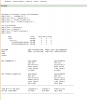2x Mirror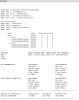3x Mirror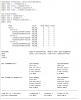so it scales ok from 1 to 2 drives, but there it gets stuck. The weird thing is that its 512 MB and 1024MB, this looks like a configured barrier

Tried to install fio on omnios but couldn't get it to work (spent only 10 mins).
Have not tried other HW changes yet... but have similar issues on another box with nvme drives (also ESX) so it seems I am doing sth fundamentally wrong

#### gea

##### Well-Known Member
Can you add a sequence without disabling all ram for caching (readcache=all)

btw
in current napp-it benchmark default is readcache=all (system default) with

Last edited:

#### gea

##### Well-Known Member
The Filebench filemicro are not very exact, they are only fast.
The filemicro read seems only testing ramcache performance not disk performance.

In current napp-it 18.01 free+, I switched Filebench defaults to
test3: randomwrite
test4: singlestreamwrite
test8: randomrw

These tests run longer but witch more exact results.
You can also compare a dd for a basic test.

If you compare other benchmarks they are mostly pure sequential without disabling RAM or enabling sync write. With Filebench you can select a lot of workload cases, each with a different result.

#### gea

##### Well-Known Member
At least the values are ok now for AiO and scale as expected up to three mirrors. Then you seem to hit a barrier. I suppose more RAM and a barebone setup can give some more performance and would show the impact of virtualisation or RAM.

#### Rand__

##### Well-Known Member
Ok, thanks for the feedback. Will try to get that done on the weekend.

#### Rand__

##### Well-Known Member
So looks like Openindiana doesn't like ES CPUs ... thought it were the MLX cards but nopeHm its not the ES CPU... still happens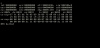Edit: It seems as if this is an USB issue with my KVM over IP switch... as soon as I plug that in ...

Will check the hint's re USB config

Last edited:

#### Rand__

##### Well-Known Member
Ok, so in the 846, 2 runs with 3 & 7 mirrors... its better than as VM, but there are still weird limits...

3 Mirrors - sync always=360MB, singlestream read 3.2 GB/s, dd 2.2 GB/s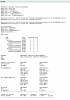7 Mirrors - sync always=360MB, singlestream read 3.2 GB/s, dd 2.2 GB/s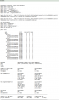#### gea

##### Well-Known Member
It is hard to go above 3 GB/s read even with faster disks.

Sync write with 300-400 MB/s seems ok (disk based pools are at 30-50 MB/s).
btw. This is quite the same with one Optane.

In my tests with 8 x Sandisk Pro Extreme 960 I achieved 150 MB/s (OmniOS)
With 4 x Optane 900P (Optane is best of all) I got 900 MB/s

On the new Solaris 11.4, I got 1200 MB/s with 4 x Optane
So I suppose if you want more you need either Optane and/or a commercial Solaris.

Last edited:

#### Rand__

##### Well-Known Member
Ok, so you say even a large number of SSDs will not get faster than this ? Now thats weird and disappointing...
Ok, I will move the physical install over to the nvme box then, got 4 P3700s waiting for tests there...